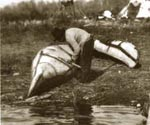Measurements: How to Create the Canoe You Envision by John Lindman

I'll try to keep this fairly simple. Write me if it is confusing.

Back in January I went over the various measurements that affect the design of a birchbark canoe. In this issue I will show you a few canoes and give the various measurements in case you wish to either copy them or make a variation from them.

Ojibwe longnose.Length = 15ft
Frame length = 12ft 3 inches
Frame width = 27 inches
Finished Beam = 34 1/2 inches
Finished Depth = 13 inches
Height stick center = 8 1/2 inches
Intermediate = 8 1/2 inches
End = 9 1/2 inches
Finished Stem height = 22 inches

14ft Tete d'boule (Attikemek)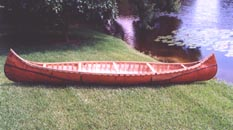Length = 14ft
Frame length = 12 ft 3 inches
Frame width = 23 inches
Finished Beam = 34 inches
Finished Depth = 13 inches
Height stick center = 9 inches
Intermediate = 9 inches
End = 10 inches
Stem height = 24 inches

17.5ft Ottawa (Ojibwe Old Style)Length = 17.5 ft
Frame length = 15ft 4 1/2 inches
Frame width = 27 inches
Finished Beam = 40 inches
Height stick center = 10 inches
Intermediate = 10 inches
End = 11 inches
Stem height = 30 inches

14ft Algonquin wabanaki chimanLength = 14 ft 3 inches
Frame length = 12 ft 3 inches
Frame width = 30 inches
Finished Beam = 34 inches
Finished Depth = 12 inches
Height stick center =7"
Intermediate = 8" second lashing past intermediate thwart
End = 17" second lashing past end thwart
Stem height = 21 inches

15ft 8in Ojibwe Longnose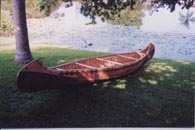Length = 15.8 ft
Frame length = 12ft 9 inches
Frame width = 30 inches
Finished Depth = 13 inches
Finished Beam = 34 inches
Height stick center = 8.5 inches
Intermediate = 8.5 inches
End = 9.5 inches
Stem height = 22 inches

14' Ojibwe Longnose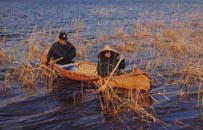Length = 14ft 3in
Frame length = 141.5 inches
Frame width = 29.25 inches
Finished Depth = 13 inches
Finished Beam = 34 inches
Height stick center = 8 inches
Intermediate = 8 inches
End = 9 inches
Stem height = 20.5 inches

15ft 9in Ojibwe Longnose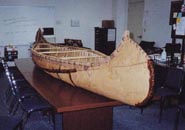Length = 15.9 ft
Frame length = 12ft 9 inches
Frame width = 30 inches
Finished Depth = 12.5 inches
Finished Beam = 34 inches
Height stick center = 8 inches
Intermediate = 8 inches
End = 9 inches
Stem height = 22 inches

Algonquin OldstyleLength = 14 ft
Frame length = 12ft 9 inches
Frame width = 30 inches
Finished Depth = 12.25 inches
Finished Beam = 34 inches
Height stick center = 7.5 inches
Intermediate = 7.5 inches
End = 8.5 inches
Stem height = 20.5 inches

Next Page

509-327-7989 voice and fax        john@barkcanoe.com e-mail